# PFR with axial dispersion - CSTR in series conversion?

• Andrew O Regan

## Homework Statement

Here is the problem description:

Develop an Excel file that given a set of data from an RTD pulse injection will determine the model parameters of the following schematics, and then predict the conversion in a CONTINUOUS reactor with a n-order reaction (where n is not equal to 1)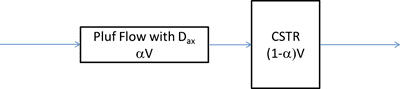## Homework Equations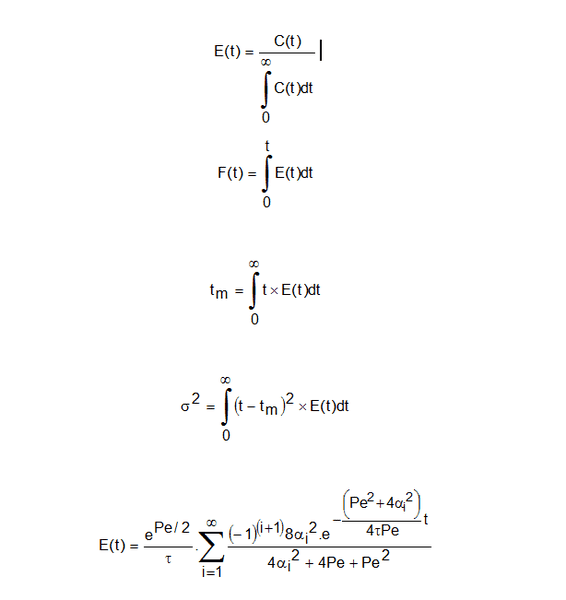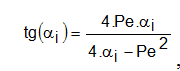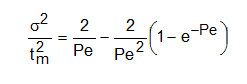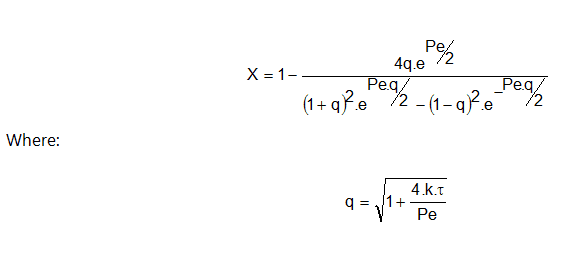## The Attempt at a Solution

The above formulae were used in this assignment to take the user inputs and populate the excel spreadsheet.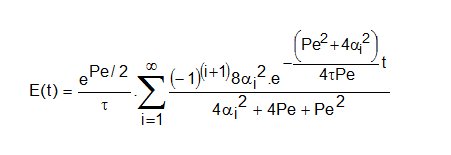The above formula is simplified to form the equation for the model which is calculated using varying values of alpha.

The flow model needs the roots of: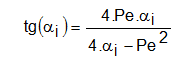Pe is calculated from the known result: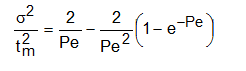This then generates the flow curve for Pe in excel.

Solving the equation above for this trial gives Pe=7.676. This was done in Excel using the Goal Seek function to find when the first term of the equation above was equal to the second.

As can be seen from the figure below, the term chosen for Pe gives a difference of 0.0152 between the terms.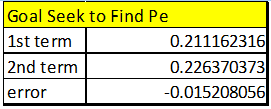To calculate the conversion, a further two equations are needed: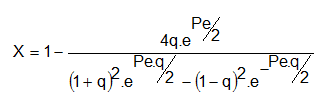Where q was calculated using formula mentioned above.

The eventual conversion calculated for this run was 68%.

This is for the conversion exiting the PFR.

The difficulty I am having is with the CSTR. I don't know how to relate the two reactors.

I am treating this setup as closed-closed.

I do not know how to translate the information I have into setting up the CSTR conversion section, to find my overall conversion?

Any information/help in guiding me to a solution would be very much appreciated.

Thank you.